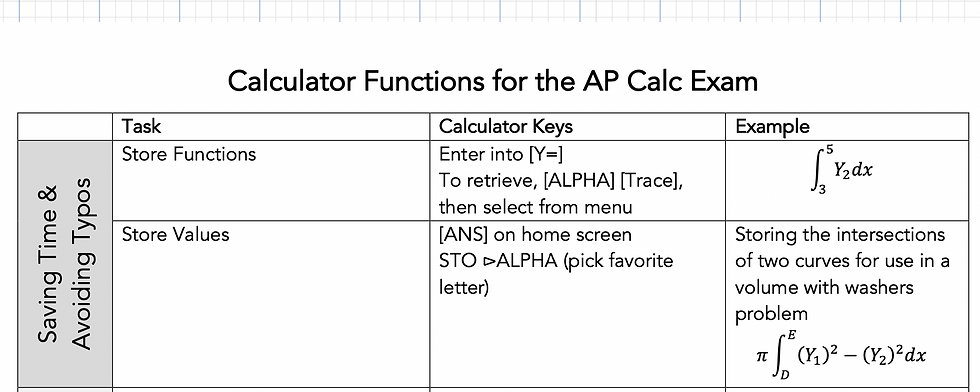top of page
Search

# How to Use Your Calculator on the AP Calc Exam

Updated: Apr 5

The AP Calculus Exam has a calculator and non-calculator section. You will be allowed to use a graphing calculator on the last 15 multiple choice questions and the first two free response questions. You can learn more about the format of the exam here.

There are a few things to keep in mind when using your calculator.

• Using a calculator does not mean you should show no work. While you don't have to do the calculations by hand, always write on your paper the equation you are solving, or the value you are evaluating, including the limits of integration when finding a definite integral.

• Save functions in your calculator as Y1, Y2, etc. instead of re-typing them each time, especially if you are using the same function multiple times (like in a Free Response Question).

• Don't round intermediate values. Keep several decimal places when finding intersection points or parameters of an equation. Final answers should be rounded to at least 3 decimal places.

• Be fluent at using your calculator to graph a function on an appropriate window, find zeros and intersections, and evaluate derivatives and integrals.

• Don't write down calculator keys as supporting justification for an answer. Use words and symbols instead. (Ex: The tangent line approximation for f(3.1) gives an overestimate because f''(3)=-2.275<0 meaning f is concave down near x=3).

If you're unsure how to do some of the items above on your calculator, fret not! We've created a guide showing you how to do a variety of calculator tasks with an example of when you might use each one.